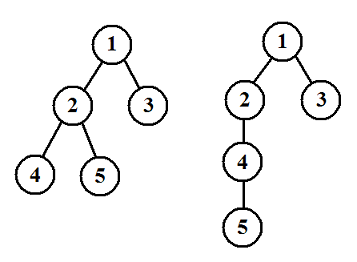# #122. 【NOI2013】树的计数\begin{equation} \frac{h_1 + h_2 + \dots + h_K}{K} \end{equation}

### 样例一

#### input

5
1 2 4 5 3
1 2 3 4 5



#### output

3.500



### 限制与约定

20% 的测试数据，满足：$n \leq 10$；

40% 的测试数据，满足：$n \leq 100$；

85% 的测试数据，满足：$n \leq 2000$；

100% 的测试数据，满足：$2 \leq n \leq 200000$。